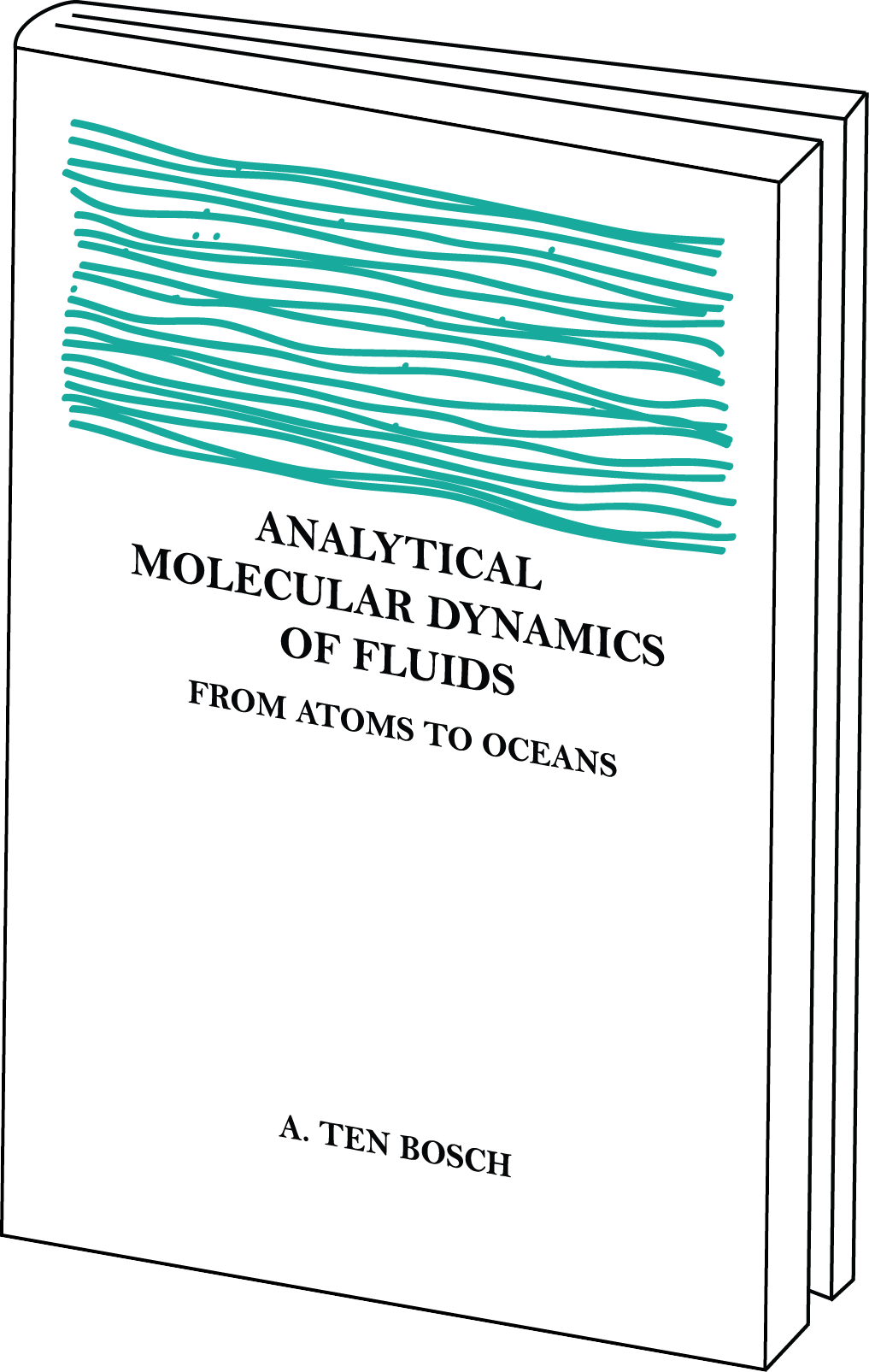• understand fluids beyond phenomenological models
• discover new concepts and techniques in fluid research
• acquire tools and background useful to solve analytical problems

# Analytical Molecular Dynamics Of Fluids: From Atoms To Oceans

A. ten Bosch

The book fills the gap between phenomenological models and modern developments and represents current research in the theory of complex fluids. The text is an outgrowth of material used by the author in graduate courses of fluids for engineering and physics students in material science.

## Contents1. Introduction to fluids
2. How to set up and solve a mathematical model
3. Equilibrium properties of fluids
4. Density Functional theory of classical fluids
5. Calculation of phase diagrams
6. Surface properties
7. Methods of fluid dynamics
8. Dynamics from microscopic to macroscopic
9. Polymer dynamics
10. Application to porous materials